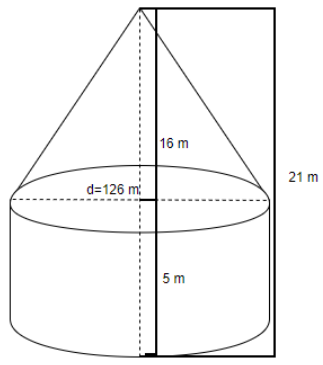QuestionAnswers

# A circus tent is made of canvas and is in the form of a right circular cylinder and a right circular cone above it. The diameter and height of the cylindrical part of the tent is 126 m and 5 m respectively. The total height of the tent is 21 m. Find the cost of canvas used to make a tent when the cost per ${{m}^{2}}$ is Rs. 15.

Hint: We have been given the diameter of the cylinder and the cone is above the cylinder. From here, we can say that the diameter of the cylinder and cone is the same. Then we can calculate the curved surface area of the tent and then multiply it with the cost of canvas per ${{m}^{2}}$ to get the total cost.

Complete step-by-step solution -
Here, we have been given that a tent is made of canvas and it is formed by placing a cone on top of a cylinder. It is also given that the height of the tent is given as 21 m, the height of the cylinder is 5 m and the diameter of the cylinder is 126 m. Now, since the cone is placed on top of the cylinder, the diameter of both the cylinder and the cone would be the same. The height of the cone can be found by subtracting the total height of the tent. So, let us draw the figure and mention the dimensions in it as below,Now, we have to calculate the curved surface area of the cylinder and cone to get the total area of the tent. For the curved surface area of the cylinder, we are using the formula:
Curved surface area of the cylinder $=2\pi rh$, where r is the radius of cylinder and h is the height of the cylinder.
We know that the diameter of the cylinder is 126 m, so the radius is $\dfrac{126}{2}=36m$ and the height of the cylinder is 5 m. We can use the value of $\pi =\dfrac{22}{7}$ . Therefore, we get the curved surface area of cylinder as:
\begin{align} & =(2\times \pi \times 63\times 5){{m}^{2}} \\ & =2\times \dfrac{22}{7}\times 63\times 5 \\ & =2\times 22\times 9\times 5 \\ & =1980{{m}^{2}}\ldots \ldots \ldots \left( i \right) \\ \end{align}
Now, we are going to calculate the curved surface area of the cone. The formula is given by:
Curved surface area of the cone $=\pi rl$, where, r is the radius of cone and l is the slant height of the cone. Slant height of the cone, $l=\sqrt{{{r}^{2}}+{{h}^{2}}}$, where h is the height of the cone.
We know that h = 21 – 5 = 16 m and r = 63 m. So, the slant height of the cone is
\begin{align} & l=\left( \sqrt{{{(63)}^{2}}+{{(16)}^{2}}} \right) \\ & l=\sqrt{3969+256} \\ & l=\sqrt{4225} \\ & l=65m \\ \end{align}
So, the curved surface area of the cone can be obtained as,
\begin{align} & =\left( \pi \times 63\times 65 \right) \\ & =\dfrac{22}{7}\times 63\times 65 \\ & =22\times 9\times 65 \\ & =12870{{m}^{2}}\ldots \ldots \ldots \left( ii \right) \\ \end{align}
Now, the curved surface area of the tent is equal to the sum of the curved surface area of the cylinder and cone. So, adding equation (i) and (ii), we get the curved surface area of tent $=(1980+12870){{m}^{2}}=14850{{m}^{2}}$.
So, cost of canvas = (curved surface area of tent in ${{m}^{2}}$) $\times$ (cost of canvas per ${{m}^{2}}$)
Since the cost of canvas per ${{m}^{2}}$ is given as Rs. 15, we can substitute it.
Cost of canvas $=Rs.\left( 14850\times 15 \right)=Rs.222750$
Hence, the cost of the canvas is Rs. 222750.

Note: In this problem, the biggest mistake is calculating the outer surface area of cylinder and cone instead of the curved surface area of cylinder and cone. For the curved surface area of the cylinder, we can also use the formula $\pi rd$, where d is the diameter of the cylinder. Another point to note is the value of $\pi$ used. Here, we have the radius as 63 which is a multiple of 7, therefore, we can use $\pi =\dfrac{22}{7}$ and get a proper answer.